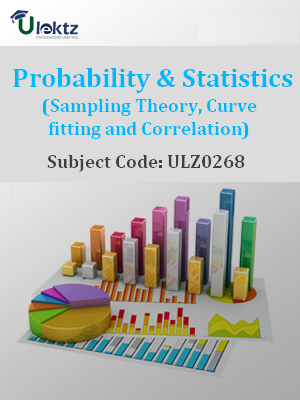•My WalletMy Order
•My Profile
•My Connections
•My Books
•My Videos
•My Tests
•My Calender
•My Messages
•My Shopping Cart
•My Orders
•Account Settings
•Help

# Book Details# Probability & Statistics (Sampling Theory, Curve fitting and Correlation)

 Course Code : ULZ0268 Author : uLektz University : General for All University Regulation : 2017 Categories : Civil Format :ePUB3 (DRM Protected) Type : eBook

FREE

Description :Probability & Statistics (Sampling Theory, Curve fitting and Correlation) of ULZ0268 covers the latest syllabus prescribed by General for All University for regulation 2017. Author: uLektz, Published by uLektz Learning Solutions Private Limited.

Note : No printed book. Only ebook. Access eBook using uLektz apps for Android, iOS and Windows Desktop PC.

##### Topics
###### UNIT I RANDOM VARIABLES AND DISTRIBUTIONS

1.1 Introduction- Random variables-Distribution function-Discrete distributions ( Review of Binomial and Poisson distributions)

1.2 Continuous distributions: Normal, Normal approximation to Binomial distribution, Gamma and Weibull distributions

###### UNIT II Moments and Generating functions

2.1 Introduction-Mathematical expectation and properties

2.2 Moment generating function-Moments of standard distributions ( Binomial, Poisson and Normal distributions) – Properties

###### UNIT III SAMPLING THEORY

3.1 Introduction - Population and samples

3.2 Sampling distribution of mean for large and small samples (with known and unknown variance)

3.3 Proportion sums and differences of means-Sampling distribution of variance -Point and interval estimators for means and proportions

###### UNIT IV TESTS OF HYPOTHESIS

4.1 Introduction - Type I and Type II errors

4.2 Maximum error - One tail, two-tail tests

4.3 Tests concerning one mean and proportion

4.4 Two means- Proportions and their differences using Z-test, Student’s t-test - F-test and Chi -square test

4.5 ANOVA for one-way and two-way classified data

###### UNIT V CURVE FITTING AND CORRELATION

5.1 Introduction - Fitting a straight line

5.2 Second degree curve-exponential curve-power curve by method of least squares

5.3 Simple Correlation and Regression

5.4 Rank correlation - Multiple regression

###### UNIT VI STATISTICAL QUALITY CONTROL METHODS

6.1 Introduction - Methods for preparing control charts

6.2 Problems using x-bar, p, R charts and attribute charts